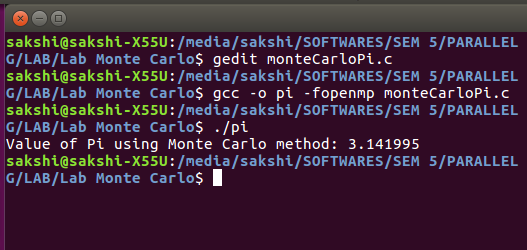Related Articles

# Estimating the value of Pi using Monte Carlo | Parallel Computing Method

• Difficulty Level : Expert
• Last Updated : 04 Oct, 2021

Given two integers N and K representing number of trials and number of total threads in parallel processing. The task is to find the estimated value of PI using the Monte Carlo algorithm using the Open Multi-processing (OpenMP) technique of parallelizing sections of the program.

Examples:

Input: N = 100000, K = 8
Output: Final Estimation of Pi = 3.146600

Input: N = 10, K = 8
Output: Final Estimation of Pi = 3.24

Input: N = 100, K = 8
Output: Final Estimation of Pi = 3.0916

Approach: The above given problem Estimating the value of Pi using Monte Carlo is already been solved using standard algorithm. Here the idea is to use parallel computing using OpenMp to solve the problem. Follow the steps below to solve the problem:

• Initialize 3 variables say x, y, and d to store the X and Y co-ordinates of a random point and the square of the distance of the random point from origin.
• Initialize 2 variables say pCircle and pSquare with values 0 to store the points lying inside circle of radius 0.5 and square of side length 1.
• Now starts the parallel processing with OpenMp together with reduction() of the following section:
• Iterate over the range [0, N] and find x and y in each iteration using srand48() and drand48() then find the square of distance of point (x, y) from origin and then if the distance is less than or equal to 1 then increment pCircle by 1.
• In each iteration of the above step, increment the count of pSquare by 1.
• Finally, after the above step calculate the value of estimated pi as below and then print the obtained value.
•  Pi = 4.0 * ((double)pCircle / (double)(pSquare))

Below is the implementation of the above approach:

## C++

 `// C++ program for the above approach``#include ``using` `namespace` `std;` `// Function to find estimated``// value of PI using Monte``// Carlo algorithm``void` `monteCarlo(``int` `N, ``int` `K)``{``  ` `    ``// Stores X and Y coordinates``    ``// of a random point``    ``double` `x, y;``  ` `    ``// Stores distance of a random``    ``// point from origin``    ``double` `d;` `    ``// Stores number of points``    ``// lying inside circle``    ``int` `pCircle = 0;` `    ``// Stores number of points``    ``// lying inside square``    ``int` `pSquare = 0;``    ``int` `i = 0;` `// Parallel calculation of random``// points lying inside a circle``#pragma omp parallel firstprivate(x, y, d, i) reduction(+ : pCircle, pSquare) num_threads(K)``    ``{``      ` `        ``// Initializes random points``        ``// with a seed``        ``srand48((``int``)``time``(NULL));` `        ``for` `(i = 0; i < N; i++)``        ``{``          ` `            ``// Finds random X co-ordinate``            ``x = (``double``)drand48();` `            ``// Finds random X co-ordinate``            ``y = (``double``)drand48();` `            ``// Finds the square of distance``            ``// of point (x, y) from origin``            ``d = ((x * x) + (y * y));` `            ``// If d is less than or``            ``// equal to 1``            ``if` `(d <= 1)``            ``{``              ` `                ``// Increment pCircle by 1``                ``pCircle++;``            ``}``          ` `            ``// Increment pSquare by 1``            ``pSquare++;``        ``}``    ``}``  ` `    ``// Stores the estimated value of PI``    ``double` `pi = 4.0 * ((``double``)pCircle / (``double``)(pSquare));` `    ``// Prints the value in pi``    ``cout << ``"Final Estimation of Pi = "``<< pi;``}` `// Driver Code``int` `main()``{``  ` `    ``// Input``    ``int` `N = 100000;``    ``int` `K = 8;``  ` `    ``// Function call``    ``monteCarlo(N, K);``}` `// This code is contributed by shivanisinghss2110`

## C

 `// C program for the above approach` `#include ``#include ``#include ``#include ` `// Function to find estimated``// value of PI using Monte``// Carlo algorithm``void` `monteCarlo(``int` `N, ``int` `K)``{``    ``// Stores X and Y coordinates``    ``// of a random point``    ``double` `x, y;``    ``// Stores distance of a random``    ``// point from origin``    ``double` `d;` `    ``// Stores number of points``    ``// lying inside circle``    ``int` `pCircle = 0;` `    ``// Stores number of points``    ``// lying inside square``    ``int` `pSquare = 0;` `    ``int` `i = 0;` `// Parallel calculation of random``// points lying inside a circle``#pragma omp parallel firstprivate(x, y, d, i) reduction(+ : pCircle, pSquare) num_threads(K)``    ``{``        ``// Initializes random points``        ``// with a seed``        ``srand48((``int``)``time``(NULL));` `        ``for` `(i = 0; i < N; i++) {``            ``// Finds random X co-ordinate``            ``x = (``double``)drand48();` `            ``// Finds random X co-ordinate``            ``y = (``double``)drand48();` `            ``// Finds the square of distance``            ``// of point (x, y) from origin``            ``d = ((x * x) + (y * y));` `            ``// If d is less than or``            ``// equal to 1``            ``if` `(d <= 1) {``                ``// Increment pCircle by 1``                ``pCircle++;``            ``}``            ``// Increment pSquare by 1``            ``pSquare++;``        ``}``    ``}``    ``// Stores the estimated value of PI``    ``double` `pi = 4.0 * ((``double``)pCircle / (``double``)(pSquare));` `    ``// Prints the value in pi``    ``printf``(``"Final Estimation of Pi = %f\n"``, pi);``}` `// Driver Code``int` `main()``{``    ``// Input``    ``int` `N = 100000;``    ``int` `K = 8;``    ``// Function call``    ``monteCarlo(N, K);``}`
Output
`Final Estimation of Pi = 3.146600`Output of above C program

Time Complexity: O(N*K)
Auxiliary Space: O(1)

Attention reader! Don’t stop learning now. Get hold of all the important mathematical concepts for competitive programming with the Essential Maths for CP Course at a student-friendly price. To complete your preparation from learning a language to DS Algo and many more,  please refer Complete Interview Preparation Course.

My Personal Notes arrow_drop_up